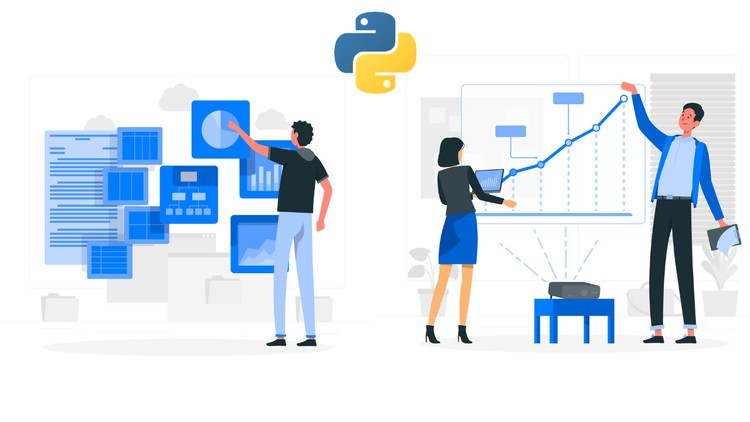# Data Visualization in Python with Covid-19 Analysis Project

Learn to use Python for Data Visualization. Practical project on applying Python for visualizing & predicting Covid-19.
How to use Python for Data Visualization
Full-fledged hands-on Project on Data Visualization with Python - "Visualizing Covid-19"
How to develop useful, intuitive and informative visualizations using Python programming
Introduction to Data Visualization - what it is, its importance & benefits
Top Python Libraries for Data Visualization
Introduction to Matplotlib, Install Matplotlib with pip
Basic Plotting with Matplotlib
NumPy and Pandas
Data Visualization tools - Bar chart, Histogram, Pie chart
More Data Visualization tools - Scatter Plot, Area Plot, Stacked Area Plot, Box Plot
Advanced data Visualization tools - Waffle Chart, Word Cloud, Heat map
Specialized data Visualization tools (I) - Bubble charts, Contour plots, Quiver Plot
Specialized data Visualization tools (II) - 3D Plotting in Matplotlib
3D Line Plot, 3 D Scatter Plot, 3D Contour Plot, 3D Wireframe Plot, 3D Surface Plot
Seaborn - Introduction to Seaborn, Seaborn functionalities, Installing Seaborn
Different categories of plot in Seaborn, Some basic plots using seaborn
Data Visualization using Seaborn - Strip Plot, Swarm Plot, Plotting Bivariate Distribution
Scatter plot, Hexbin plot, KDE, Regplot, Visualizing Pairwise Relationship, Box plot, Violin Plots, Point Plot

Data Visualization with Python – course syllabus

1. Introduction to Data Visualization

• What is data visualization

• Benefits of data visualization

• Importance of data visualization

• Top Python Libraries for Data Visualization

2. Matplotlib

• Introduction to Matplotlib

• Install Matplotlib with pip

• Basic Plotting with Matplotlib

• Plotting two or more lines on the same plot

3. Numpy and Pandas

• What is numpy?

• Why use numpy?

• Installation of numpy

• Example of numpy

• What is a panda?

• Key features of pandas

• Python Pandas – Environment Setup

• Pandas – Data Structure with example

4. Data Visualization tools

• Bar chart

• Histogram

• Pie Chart

5. More Data Visualization tools

• Scatter Plot

• Area Plot

• STACKED Area Plot

• Box Plot

• Waffle Chart

• Word Cloud

• HEAT MAP

7. Specialized data Visualization tools (Part-I)

• Bubble charts

• Contour plots

• Quiver Plot

8. Specialized data Visualization tools (Part-II)

Three-Dimensional Plotting in Matplotlib

• 3D Line Plot

• 3D Scatter Plot

• 3D Contour Plot

• 3D Wireframe Plot

• 3D Surface Plot

9. Seaborn

• Introduction to seaborn

• Seaborn Functionalities

• Installing seaborn

• Different categories of plot in Seaborn

• Some basic plots using seaborn

10. Data Visualization using Seaborn

• Strip Plot

• Swarm Plot

• Plotting Bivariate Distribution

• Scatter plot, Hexbin plot, KDE, Regplot

• Visualizing Pairwise Relationship

• Box plot, Violin Plots, Point Plot

11. Project on Data Visualization

### Introduction to Data Visualization

1
Introduction to Data Visualisation

### Matplotlib

1
Matplotlib - part 1
2
Matplotlib - part 2

### NumPy and Pandas

1
NumPy and Pandas - part 1
2
NumPy and Pandas - part 2
3
NumPy and Pandas - part 3

### Data Visualization Tools

1
Data Visualisation Tools - part 1
2
Data Visualisation Tools - part 2
3
Data Visualisation Tools - part 3

### More Data Visualization Tools

1
More Data Visualisation Tools - part 1
2
More Data Visualisation Tools - part 2
3
More Data Visualisation Tools - part 3

1
Advanced Data Visualisation Tools - part 1
2
Advanced Data Visualisation Tools - part 2
3
Advanced Data Visualisation Tools - part 3

### Specialized Data Visualization Tools (I)

1
Specialized Data Visualisation Tools (I) - part 1
2
Specialized Data Visualisation Tools (I) - part 2
3
Specialized Data Visualisation Tools (I) - part 3

### Specialized Data Visualization Tools (II)

1
Specialized Data Visualisation Tools (II) - part 1
2
Specialized Data Visualisation Tools (II) - part 2
3
Specialized Data Visualisation Tools (II) - part 3
4
Specialized Data Visualisation Tools (II) - part 4

1
Seaborn - part 1
2
Seaborn - part 2
3
Seaborn - part 3

### Data Visualization using Seaborn

1
Data Visualisation using Seaborn - part 1
2
Data Visualisation using Seaborn - part 2
3
Data Visualisation using Seaborn - part 3
4
Data Visualisation using Seaborn - part 4
5
Data Visualisation using Seaborn - part 5

### Project on Data Visualization with Python

1
Project on Data Visualisation with Python - part 1
2
Project on Data Visualisation with Python - part 2
3
Project on Data Visualisation with Python - part 3
4
Project on Data Visualisation with Python - part 4
5
Project on Data Visualisation with Python - part 5
6
Project on Data Visualisation with Python - part 6
You can view and review the lecture materials indefinitely, like an on-demand channel.
Definitely! If you have an internet connection, courses on Udemy are available on any device at any time. If you don't have an internet connection, some instructors also let their students download course lectures. That's up to the instructor though, so make sure you get on their good side!

#### Be the first to add a review.